Question

Which reactant is being oxidized in the following reaction?

Cl2 + 2 KI --> 2 KCl + I2
Just give the reactant. No need to include the coefficient if there is one.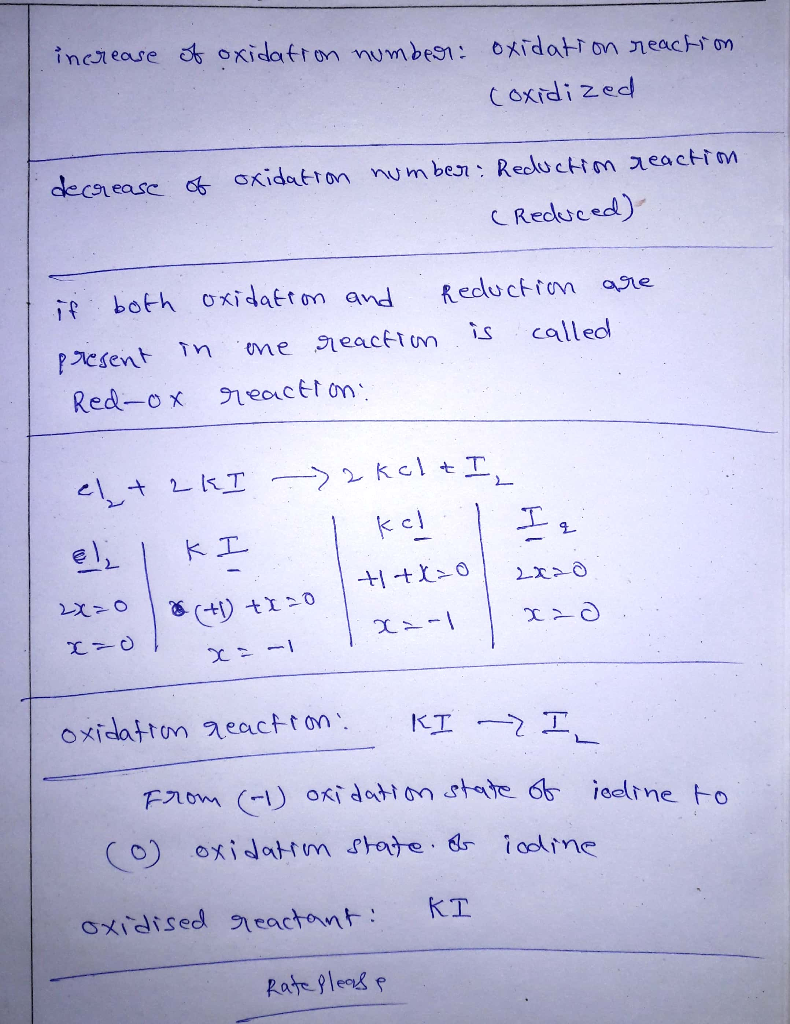#### Earn Coins

Coins can be redeemed for fabulous gifts.

Similar Homework Help Questions
• ### In each of the following reactions, identify the reactant that is oxidized and the reactant that...

In each of the following reactions, identify the reactant that is oxidized and the reactant that is reduced: (its multiple choice) Number 1: Br2(g)+2KI(aq)→2KBr(aq)+I2(s) I− (in KI) loses electrons and is oxidized. Br2 gains electrons and is reduced. Br2 gains electrons and is oxidized. I− (in KI) loses electrons and is reduced. I− (in KI) gains electrons and is oxidized. Br2 loses electrons and is reduced. Br2 loses electrons and is oxidized. I− (in KI) gains electrons and is reduced....

• ### 1)Balance the following chemical reaction, if necessary. Identify the reactant that is Oxidized and the reactant th...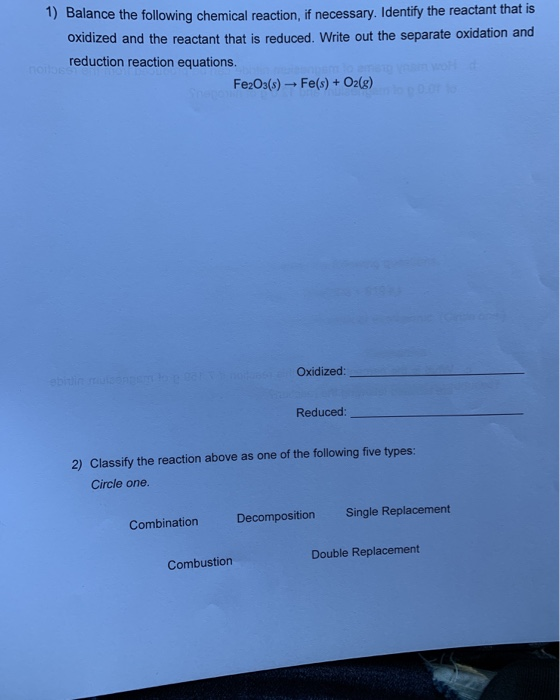1)Balance the following chemical reaction, if necessary. Identify the reactant that is Oxidized and the reactant that is reduced. Write out the separate oxidation and reduction reaction equations. noilbse Fe2O03(s)- Fe(s) + O2(g) Speco Oxidized: Reduced: 2) Classify the reaction above as one of the following five types: Circle one. Single Replacement Decomposition Combination Double Replacement Combustion

• ### For each reaction below, identify which reactant is oxidized and which is reduced. a) 2 Fe3+...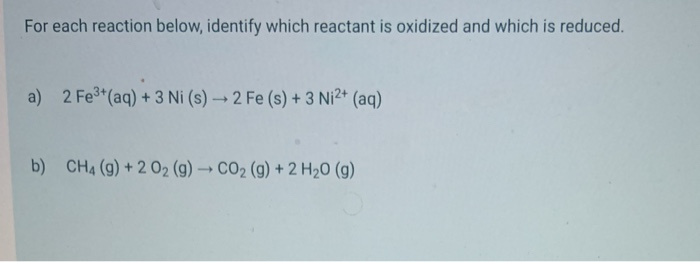For each reaction below, identify which reactant is oxidized and which is reduced. a) 2 Fe3+ (aq) + 3 Ni (s) - 2 Fe (s) + 3 Ni2+ (aq) b) CH4 (9) + 2 O2 (g) - CO2 (g) + 2 H20 (9)

• ### For each chemical reaction listed in the table below, decide whether the highlighted atom is being oxidized or reduced.

For each chemical reaction listed in the table below, decide whether the highlighted atom is being oxidized or reduced. reactionNa₂ CO₃(s)+H₃ PO₄(aq) → Na₂ HPO₄(aq)+CO₂(g)+H₂ O(l) 4 HF(g)+SiO₂(s) → SiF₄(g)+2 H₂ O(g) 2 H₂(g)+O₂(g) → 2 H₂ O(g) 4 KI(aq)+2 CuCl₂(aq) → 2 CuI(s)+I₂(aq)+4 KCl(aq)

• ### Identify which element is being reduced and oxidized in the following reaction. (For full credit, please...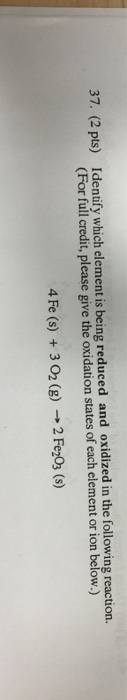Identify which element is being reduced and oxidized in the following reaction. (For full credit, please give the oxidation states of each clement or ion below.) 4 Fe (s) + 3 O_2(g) rightarrow 2Fe_2Q_3 (s)

• ### Which of the following elements is being oxidized in the reaction below? Cu (s) + 2AgNO3...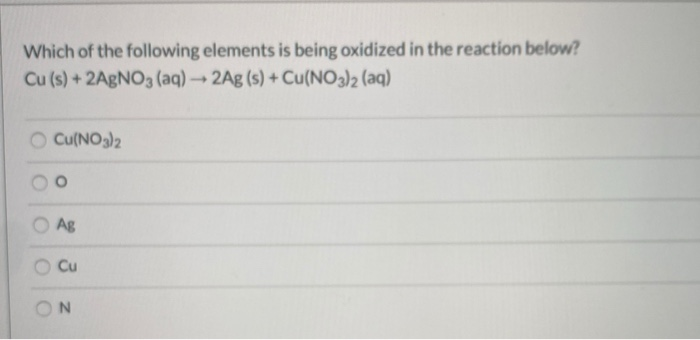Which of the following elements is being oxidized in the reaction below? Cu (s) + 2AgNO3 (aq) + 2Ag (s) + Cu(NO3)2 (aq) Cu(NO3)2 Ag o Cu ON

• ### Select the oxidation-reduction reaction(s)? 1. Pb(ClO4)2(aq) + 2 KI(aq) → PbI2(s) + 2 KClO4(aq) 2. Cl2(g)...

Select the oxidation-reduction reaction(s)? 1. Pb(ClO4)2(aq) + 2 KI(aq) → PbI2(s) + 2 KClO4(aq) 2. Cl2(g) + CuI2(aq) → CuCl2(aq) + I2(aq) 3. CaCO3(s) → CO2(g) + CaO(s) Group of answer choices 1 only 3 only 2 only 1 and 3

• ### Redox Equations Which of the following reactions are redox reactions? Identify the substance oxidized, the substance...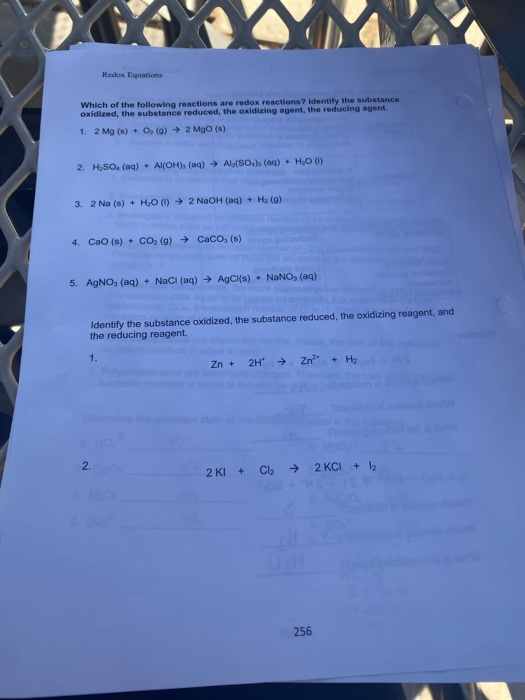Redox Equations Which of the following reactions are redox reactions? Identify the substance oxidized, the substance reduced, the oxidizing agent, the reducing agent. 1.2 Mg(s) + O2(g) → 2 MgO (s) 2. H SO. (aq) + Al(OH) (aq) → Al(SO.) (aq) + H,O (1) 3. 2 Na (s) + H2O (1) ► 2 NaOH(aq) + H2(g) 4. Cao (s) + CO2(g) → Caco, (s) 5. AgNO, (aq) + NaCl (aq) ► AgCl(s) + NaNO, (aq) Identify the substance oxidized, the...

• ### 2. This chemical reaction is an oxidation reduction reaction. a. What is being oxidized in this...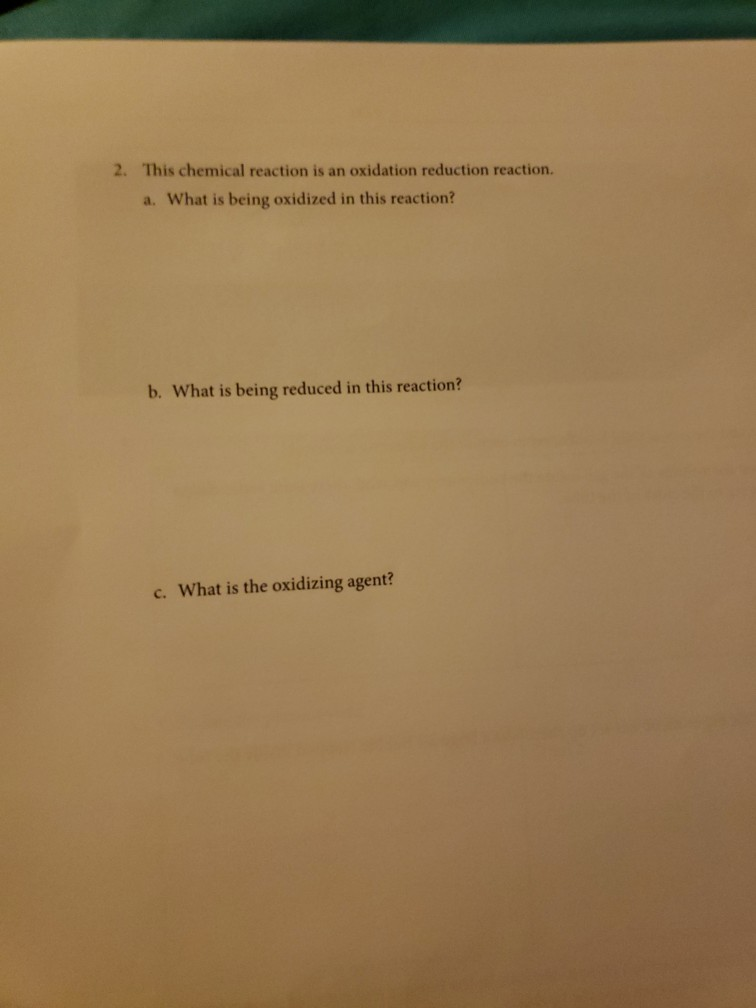2. This chemical reaction is an oxidation reduction reaction. a. What is being oxidized in this reaction? b. What is being reduced in this reaction? c. What is the oxidizing agent?

• ### 1. Identify the species being oxidized and reduced in each of the following reactions, and determine...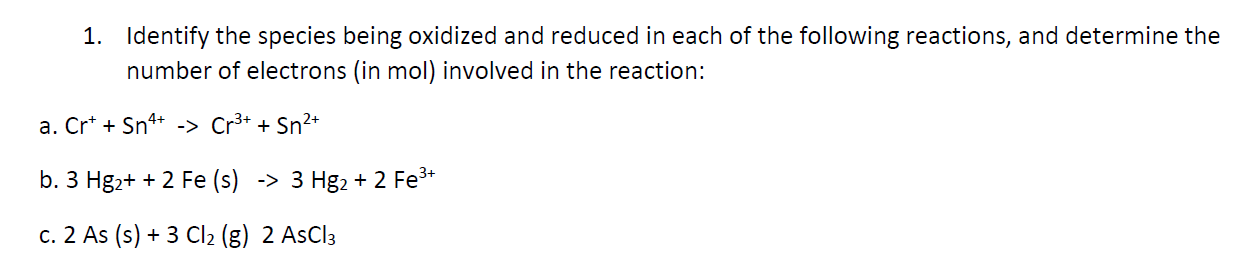1. Identify the species being oxidized and reduced in each of the following reactions, and determine the number of electrons (in mol) involved in the reaction: a. Crt + Sn4+ -> Cr3+ + Sn2+ b. 3 Hg2+ + 2 Fe (s) -> 3 Hg2+ 2 Fe3+ c. 2 As (s) + 3 Cl2 (8) 2 AsCl3